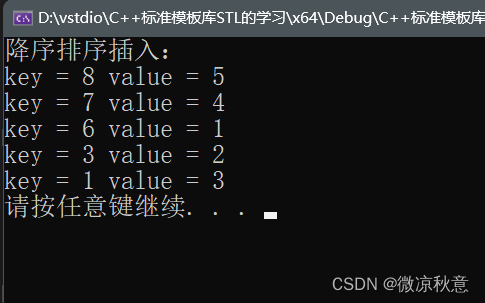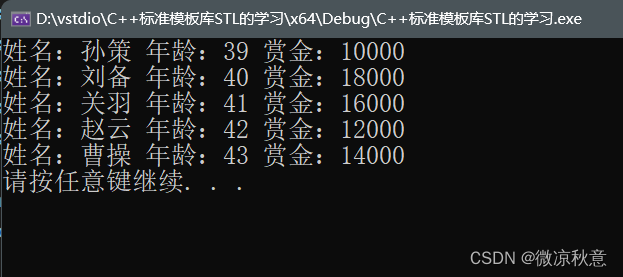> 文档中心 > ＜C++＞map 容器快速上手|自定义数据类型排序的避坑理解

# ＜C++＞map 容器快速上手|自定义数据类型排序的避坑理解

✅作者简介：热爱后端语言的大学生，CSDN内容合伙人
✨精品专栏：C++面向对象
🔥系列专栏：C++泛型编程

### 文章目录

• 1、map 容器基本操作，从构造到查找统计
• 1.1、map/ multimap 基本概念
• 1.2、map 赋值和构造
• 1.3、map 大小和交换
• 1.4、map 插入和删除
• 1.5、map 查找和统计
• 2、自定义排序规则
• 2.1、内置数据类型的排序
• 2.2、自定义数据类型的排序

🔥前言

`set` 容器后，今天总结一下 `map` 容器的功能，从零到一快速掌握基本使用与常用接口。`map``STL` 编程中与 `vector``list``set` 具有同等重要的地位，键值对的方式存储元素在查找时很是高效，那么下面正式开始 `map` 容器的学习之旅。

# 1、map 容器基本操作，从构造到查找统计

## 1.1、map/ multimap 基本概念

• map中所有元素都是二元组`pair`
• 二元组中第一个元素为`key`（键），起到索引作用，第二个元素为`value`（值）
• 优点：可以根据key值快速找到value值
• 所有元素都会根据元素的键值自动升序排序

• map/multimap属于关联式容器，底层结构是用二叉树实现

• `map`不允许容器中有重复key值元素
• `multimap`允许容器中有重复key值元素

## 1.2、map 赋值和构造

• 对map容器进行构造和赋值操作

• `map mp;` 默认构造函数:
• `map(const map &mp);` 拷贝构造函数
• `map& operator=(const map &mp);` 重载等号操作符

``// 遍历容器void printInfo(map<int,int>& mp){for (map<int, int>::iterator it = mp.begin(); it != mp.end(); it++) {cout << "key = " << it->first << " value = " << it->second << endl;}cout << endl;}// 构造和赋值void test01(){// 默认构造map<int, int> mp1;// 利用二元组显示创建mp1.insert(make_pair(1,1));mp1.insert(make_pair(5,2));mp1.insert(make_pair(4,5));printInfo(mp1);// 拷贝构造map<int, int> mp2(mp1);printInfo(mp2);// 赋值map<int, int> mp3;mp3 = mp2;printInfo(mp3);}``

## 1.3、map 大小和交换

• 统计map容器大小以及交换map容器

• `size();` 返回容器中元素的数目
• `empty();` 判断容器是否为空
• `swap(st);` 交换两个集合容器

``// 大小和交换void test02(){// 大小map<int, int>mp;mp.insert(pair<int, int>(1, 10));mp.insert(pair<int, int>(3, 30));mp.insert(pair<int, int>(2, 20));if (mp.empty()){cout << "m为空" << endl;}else{cout << "m的大小为： " << mp.size() << endl;}// 交换map<int, int>m2;m2.insert(pair<int, int>(4, 100));m2.insert(pair<int, int>(5, 200));m2.insert(pair<int, int>(6, 300));cout << "交换前" << endl;printInfo(mp);printInfo(m2);cout << "交换后" << endl;mp.swap(m2);printInfo(mp);printInfo(m2);}``

## 1.4、map 插入和删除

• map容器进行插入数据和删除数据

• `insert(elem);` 在容器中插入元素。
• `clear();` 清除所有元素
• `erase(pos);` 删除pos迭代器所指的元素，返回下一个元素的迭代器。
• `erase(beg, end);` 删除区间[beg,end)的所有元素 ，返回下一个元素的迭代器。
• `erase(key);` 删除容器中值为key的元素。

``// 插入和删除void test03(){//插入操作map<int, int> mp;//第一种插入方式mp.insert(pair<int, int>(1, 10));//第二种插入方式，推荐mp.insert(make_pair(2, 20));//第三种插入方式mp.insert(map<int, int>::value_type(3, 30));//第四种插入方式，不推荐：当不存在此值就会自动创建键，值为0mp = 40;// 模拟误操作cout << "下标为6的值为："<<mp << endl;printInfo(mp);//删除，按迭代器mp.erase(mp.begin());printInfo(mp);// 删除，按下标mp.erase(3);mp.erase(6);printInfo(mp);//清空mp.erase(mp.begin(), mp.end());mp.clear();printInfo(mp);}``

## 1.5、map 查找和统计

• 对map容器进行查找数据以及统计数据

• `find(key);` 查找key是否存在,若存在，返回该键对应的迭代器；若不存在，返回`end()`;
• `count(key);` 统计key的元素个数

``// 查找和统计void test04(){map<int, int>mp;mp.insert(pair<int, int>(1, 10));mp.insert(pair<int, int>(2, 20));mp.insert(pair<int, int>(4, 40));mp.insert(pair<int, int>(3, 30));//查找map<int, int>::iterator pos = mp.find(3);if (pos != mp.end()){cout << "找到了元素 key = " << (*pos).first << " value = " << (*pos).second << endl;}else{cout << "未找到元素" << endl;}//统计int num = mp.count(2);cout << "num = " << num << endl;}``

# 2、自定义排序规则

`set` 容器一样，定义排序规则需要在创建容器的时候就指定，同样需要借助仿函数，也就是后面会提到的 谓词

## 2.1、内置数据类型的排序

``// 内置数据类型排序class descmp{public:bool operator()(int val1, int val2) const {    // 指定为降序排列 return val1 > val2;}};void test05(){cout << "降序排序插入：" << endl;map<int, int,descmp> mp;mp.insert(make_pair(3, 2));mp.insert(make_pair(7, 4));mp.insert(make_pair(6, 1));mp.insert(make_pair(8, 5));mp.insert(make_pair(1, 3));for (auto it = mp.begin(); it != mp.end(); it++) {cout << "key = " << it->first << " value = " << it->second << endl;}}``## 2.2、自定义数据类型的排序

``// 自定义数据类型排序class Hero{public:// 构造方法Hero(string name, int age) :name(name), age(age) {}// 属性string name;int age;};class Cmp_hero{public:bool  operator()(const Hero& h1, const Hero& h2)const {return h1.age < h2.age;}};void test06(){map<Hero, int, Cmp_hero> mh;Hero h1("赵云", 42);Hero h2("曹操", 43);Hero h3("孙策", 39);Hero h4("刘备", 40);Hero h5("关羽", 41);mh.insert(make_pair(h1, 12000));mh.insert(make_pair(h2, 14000));mh.insert(make_pair(h3, 10000));mh.insert(make_pair(h4, 18000));mh.insert(make_pair(h5, 16000));for (auto t = mh.begin(); t != mh.end(); t++) {cout << "姓名：" << t->first.name << " 年龄：" << t->first.age<< " 赏金：" << t->second << endl;}}```map ` 容器键值对存储的使用是非常广泛的，下篇博客来做一个员工分组的具体案例，巩固一些 `STL`常用容器的使用，大家可以订阅专栏，方便查阅和复习。